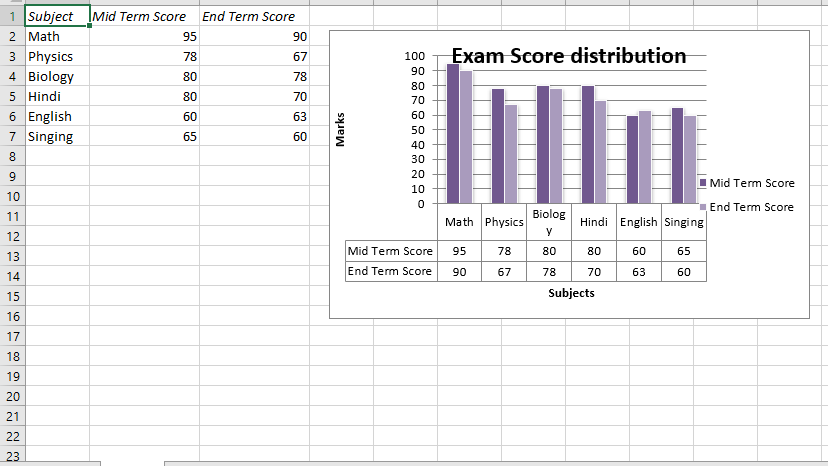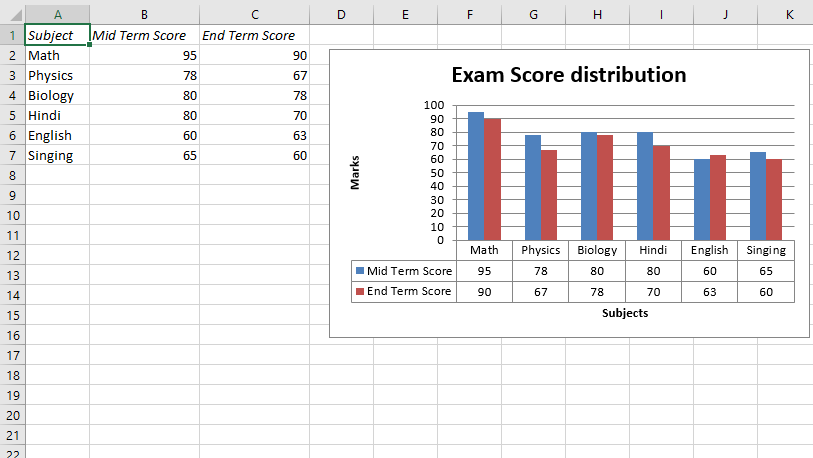GFG App
Open AppBrowser
Continue

# Python | Plotting column charts in excel sheet with data tables using XlsxWriter module

Prerequisite: Create and Write on an excel sheet
XlsxWriter is a Python library using which one can perform multiple operations on excel files like creating, writing, arithmetic operations and plotting graphs. Let’s see how to plot charts with Data tables using realtime data.
Charts are composed of at least one series of one or more data points. Series themselves are comprised of references to cell ranges. For plotting the charts on an excel sheet, firstly, create chart object of specific chart type( i.e Column chart etc.). After creating chart objects, insert data in it and lastly, add that chart object in the sheet object.

Code #1 : Plot a column chart with default data table.

For plotting this type of chart on an excel sheet, use set_table() method of the chart object.

## Python3

 `# import xlsxwriter module ` `import` `xlsxwriter`   `# Workbook() takes one, non-optional, argument   ` `# which is the filename that we want to create. ` `workbook ``=` `xlsxwriter.Workbook(``'Ex_chart2.xlsx'``)`   `# The workbook object is then used to add new   ` `# worksheet via the add_worksheet() method.` `worksheet ``=` `workbook.add_worksheet()`   `# Create a new Format object to formats cells ` `# in worksheets using add_format() method . ` `  `  `# here we create italic format object ` `bold ``=` `workbook.add_format({``'italic'``: ``1``})`   `# Add the worksheet data that the charts will refer to.` `Data1 ``=` `[``'Subject'``, ``'Mid Term Score'``, ``'End Term Score'``]` `Data2 ``=` `[[``"Math"``, ``"Physics"``, ``"Biology"``, ``"Hindi"``, ``"English"``, ``"Singing"``],` `         ``[``95``, ``78``, ``80``, ``80``, ``60``, ``65``],` `         ``[``90``, ``67``, ``78``, ``70``, ``63``, ``60``]]`   `# Write a row of data starting from 'A1' ` `# with bold format .` `worksheet.write_row(``'A1'``, Data1, bold)`   `# Write a column of data starting from  ` `# 'A2', 'B2', 'C2' respectively . ` `worksheet.write_column(``'A2'``, Data2[``0``])` `worksheet.write_column(``'B2'``, Data2[``1``])` `worksheet.write_column(``'C2'``, Data2[``2``])`   `# set the width of B and C column` `worksheet.set_column(``'B:C'``, ``15``)`   `# Create a chart object that can be added ` `# to a worksheet using add_chart() method. `   `# here we create a column chart object . ` `chart1 ``=` `workbook.add_chart({``'type'``: ``'column'``})`   `# Add a data series to a chart ` `# using add_series method. ` `  `  `# Configure the first series. ` `# = Sheet1 !\$A\$1 is equivalent to ['Sheet1', 0, 0].`   `# note : spaces is not inserted in b/w` `# = and Sheet1, Sheet1 and !` `# if space is inserted it throws warning.` `chart1.add_series({` `    ``'name'``:       ``'= Sheet1 !\$B\$1'``,` `    ``'categories'``: ``'= Sheet1 !\$A\$2:\$A\$7'``,` `    ``'values'``:     ``'= Sheet1 !\$B\$2:\$B\$7'``, })`   `# Configure a second series. ` `# Note use of alternative syntax to define ranges. ` `# [sheetname, first_row, first_col, last_row, last_col]. ` `chart1.add_series({` `    ``'name'``:       [``'Sheet1'``, ``0``, ``2``],` `    ``'categories'``: [``'Sheet1'``, ``1``, ``0``, ``6``, ``0``],` `    ``'values'``:     [``'Sheet1'``, ``1``, ``2``, ``6``, ``2``], })`   `# Add a chart title  ` `chart1.set_title({``'name'``: ``'Exam Score distribution'``})`   `# Add x-axis label ` `chart1.set_x_axis({``'name'``: ``'Subjects'``})`   `# Add y-axis label ` `chart1.set_y_axis({``'name'``: ``'Marks'``})`   `# set the style of the chart.` `chart1.set_style(``14``)`   `# set the plot area layout of chart` `chart1.set_plotarea({` `    ``'layout'``: {` `        ``'x'``:      ``0.15``,` `        ``'y'``:      ``0.09``,` `        ``'width'``:  ``0.63``,` `        ``'height'``: ``0.40``, } })`   `# Set a data table on the X-Axis` `# with the legend keys shown.` `chart1.set_table()`   `# add chart to the worksheet with given` `# offset values at the top-left corner of` `# a chart is anchored to cell D2 .` `worksheet.insert_chart(``'D2'``, chart1, ` `    ``{``'x_offset'``: ``20``, ``'y_offset'``: ``5``})`   `# Finally, close the Excel file  ` `# via the close() method.  ` `workbook.close()`

Output :Code #2 : Plot a column chart with default data table with legend keys.
For plotting this type of chart on an excel sheet, use set_table() method with ‘show_keys’ keyword argument of the chart object.

## Python3

 `# import xlsxwriter module ` `import` `xlsxwriter`   `# Workbook() takes one, non-optional, argument   ` `# which is the filename that we want to create. ` `workbook ``=` `xlsxwriter.Workbook(``'Ex_chart1.xlsx'``)`   `# The workbook object is then used to add new   ` `# worksheet via the add_worksheet() method.` `worksheet ``=` `workbook.add_worksheet()`   `# Create a new Format object to formats cells ` `# in worksheets using add_format() method . ` `  `  `# here we create italic format object ` `bold ``=` `workbook.add_format({``'italic'``: ``1``})`   `# Add the worksheet data that the charts will refer to.` `Data1 ``=` `[``'Subject'``, ``'Mid Term Score'``, ``'End Term Score'``]` `Data2 ``=` `[ [``"Math"``, ``"Physics"``, ``"Biology"``, ``"Hindi"``, ``"English"``, ``"Singing"``],` `          ``[``95``, ``78``, ``80``, ``80``, ``60``, ``65``],` `          ``[``90``, ``67``, ``78``, ``70``, ``63``, ``60``] ]`   `# Write a row of data starting from 'A1' ` `# with bold format .` `worksheet.write_row(``'A1'``, Data1, bold)`   `# Write a column of data starting from  ` `# 'A2', 'B2', 'C2' respectively . ` `worksheet.write_column(``'A2'``, Data2[``0``])` `worksheet.write_column(``'B2'``, Data2[``1``])` `worksheet.write_column(``'C2'``, Data2[``2``])`   `# set the width of B and C column` `worksheet.set_column(``'B:C'``, ``15``)`   `# Create a chart object that can be added ` `# to a worksheet using add_chart() method. `   `# here we create a column chart object . ` `chart2 ``=` `workbook.add_chart({``'type'``: ``'column'``})`   `# Add a data series to a chart ` `# using add_series method. ` `  `  `# Configure the first series. ` `# = Sheet1 !\$A\$1 is equivalent` `# to ['Sheet1', 0, 0].`   `# note : spaces is not inserted in b / w` `# = and Sheet1, Sheet1 and !` `# if space is inserted it throws warning.` `chart2.add_series({` `    ``'name'``:       ``'= Sheet1 !\$B\$1'``,` `    ``'categories'``: ``'= Sheet1 !\$A\$2:\$A\$7'``,` `    ``'values'``:     ``'= Sheet1 !\$B\$2:\$B\$7'``, })`   `# Configure a second series. ` `# Note use of alternative syntax to define ranges. ` `# [sheetname, first_row, first_col, last_row, last_col]. ` `chart2.add_series({` `    ``'name'``:       [``'Sheet1'``, ``0``, ``2``],` `    ``'categories'``: [``'Sheet1'``, ``1``, ``0``, ``6``, ``0``],` `    ``'values'``:     [``'Sheet1'``, ``1``, ``2``, ``6``, ``2``], })`   `# Add a chart title  ` `chart2.set_title({``'name'``: ``'Exam Score distribution'``})`   `# Add x-axis label ` `chart2.set_x_axis({``'name'``: ``'Subjects'``})`   `# Add y-axis label ` `chart2.set_y_axis({``'name'``: ``'Marks'``})`   `# Set a data table on the X-Axis with the legend keys shown.` `chart2.set_table({``'show_keys'``: ``True``})`   `# hide the chart legends.` `chart2.set_legend({``'position'``: ``'none'``})`   `# add chart to the worksheet with given` `# offset values at the top-left corner of` `# a chart is anchored to cell D2 .` `worksheet.insert_chart(``'D2'``, chart2,` `     ``{``'x_offset'``: ``20``, ``'y_offset'``: ``5``})`   `# Finally, close the Excel file  ` `# via the close() method.  ` `workbook.close()`

Output :My Personal Notes arrow_drop_up